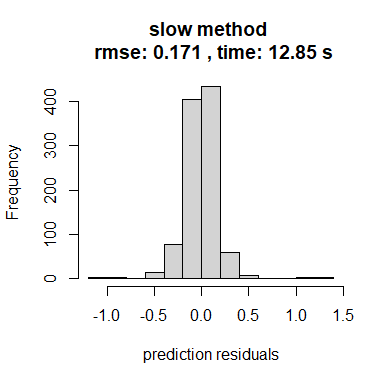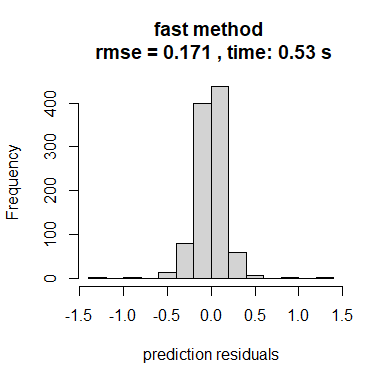# Speeding up inference

This page is designed to explain how outerbase can facilitate fast inference with smart modeling choices.

library(outerbase)

The potential benefits grow as the sample size grows. We use a sample size of 500 here in the spirit of running quickly. The point will be obvious, but more dramatic results can be had by increasing the sample size.

sampsize = 500
d = 8
x = matrix(runif(sampsize*d),ncol=d)
y = obtest_borehole8d(x)

First setup an outermod object.

om = new(outermod)
setcovfs(om, rep("mat25pow",8))
knotlist = list();
for(k in 1:d) knotlist[[k]] = seq(0.01,1,by=0.025)
setknot(om, knotlist) #40 knot point for each dim

More data should mean more basis functions. So we will choose 250 terms for our feature space approximation.

p = 250
terms = om$selectterms(p) ## Different models To begin, lets use ?loglik_std to represent our slow approach. loglik_slow = new(loglik_std, om, terms, y, x) logpr_slow = new(logpr_gauss, om, terms) logpdf_slow = new(lpdfvec, loglik_slow, logpr_slow) logpdf_slow can be optimized using lpdf$optnewton.

logpdf_slow$optnewton() Newton’s method involves solving a linear system, thus it takes one step, but is expensive. ?loglik_gauss is a lpdf model designed for speed. It is a nice comparison because loglik_gauss uses the same model as loglik_std, with a few approximations for speed. loglik_fast = new(loglik_gauss, om, terms, y, x) logpr_fast = new(logpr_gauss, om, terms) logpdf_fast = new(lpdfvec, loglik_fast, logpr_fast) logpdf_fast will through an error if you try to use optnewton. This is because it is written so that it never builds a Hessian (hess in the code) matrix. logpdf_fast$optnewton()
#> Error in logpdf_fast$optnewton(): addition: incompatible matrix dimensions: 0x0 and 250x250 It is instead suggested to use lpdf$optcg (conjugate gradient) to optimize the coefficients in the fast version.

logpdf_fast$optcg(0.001, # tolerance 100) # max epochs As an aside, omp speed ups are possible, but you need to have correctly compiled with omp. One check is to call the following. ob = new(outerbase, om, x) ob$nthreads
#>  4

If the answer is 1 but you have a multicore processor (most modern processors), your installation might be incorrect.

You can manually set the number of threads for lpdf objects.

logpdf_slow$setnthreads(4) logpdf_fast$setnthreads(4)

## Timing

The main cost of fitting outerbase models is hyperparameter optimization. The difference between logpdf_slow and logpdf_fast will be apparent. Let’s save starting points (since they share om) for fairness.

parlist_slow = list(para = getpara(logpdf_slow), hyp = gethyp(om))
parlist_fast = list(para = getpara(logpdf_fast), hyp = gethyp(om))

Test points will verify the predictions are equally good with either model, the only difference is speed.

xtest = matrix(runif(1000*d),ncol=d) #prediction points
ytest =  obtest_borehole8d(xtest)

We will use the unsophisticated proc.time to do some quick timing comparisons.

ptm = proc.time()
opth = BFGS_lpdf(om, logpdf_slow,
parlist=parlist_slow, newt=TRUE)
t_slow = proc.time() - ptm
pred_slow = new(predictor,loglik_slow)
pred_slow$update(xtest) yhat_slow = as.vector(pred_slow$mean())
print(t_slow)
#>    user  system elapsed
#>   13.36    0.20   12.85
ptm = proc.time()
opth = BFGS_lpdf(om, logpdf_fast,
parlist=parlist_fast, newt=FALSE)
t_fast = proc.time() - ptm
pred_fast = new(predictor,loglik_fast)
pred_fast$update(xtest) yhat_fast = as.vector(pred_fast$mean())
print(t_fast)
#>    user  system elapsed
#>    1.52    0.03    0.53

## Comparison of results

And simply plotting the results tells the story: faster inference with no discernible drop off in quality. Note there are serious approximations here, but the approximations just have a negligible effect.

rmse_slow = sqrt(mean((ytest-yhat_slow)^2))
hist((ytest-yhat_slow), main=paste("slow method \n rmse:",
round(rmse_slow,3),
", time:",
round(t_slow,2),'s'),
xlab = "prediction residuals")
rmse_fast = sqrt(mean((ytest-yhat_fast)^2))
hist((ytest-yhat_fast), main=paste("fast method \n rmse =",
round(rmse_fast,3),
", time:",
round(t_fast,2),'s'),
xlab = "prediction residuals")